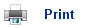# The Alkanes

• Alkanes are saturated hydrocarbons; they only contain single bonds. They are called saturated because they contain the maximum amount of hydrogen atoms possible.
• The general formula for an alkane is CnH2n+2 where n is a positive integer.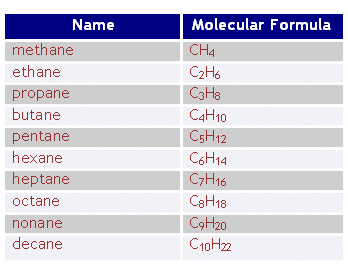• Above is a table with the first ten alkanes. Note that at the end of each name is “-ane” this is the same for all alkanes. At the beginning of the word is the stem “meth-“, “eth-“….. this relates to how many carbons there are in the alkane.
• A series of compounds that are all related, like alkanes, are called a homologous series.
• All the series have the same general formula, but each member differs from the next by a CH2 unit.
• All the compounds in the series have similar chemical properties, but different physical properties such as boiling point and density. However the physical properties change gradually in the series as the number of carbons increase.

## Alkane structural Isomers

• As well has just having a straight line chained carbons, it is often possible for branches of chains to branch off the primary chain.
• There are often many different structural formulas for a given molecular formula.

## For Example:

C4H10 has two possible structural formulae: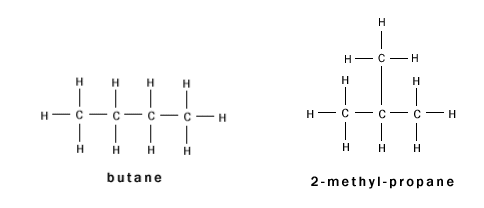• These two compounds are isomers, because they have the same chemical formula, but different structural formulae.
• Notice that the branched alkane has a different name; it is considered to have been formed from a propane group with an added methyl group. It is therefore called methyl propane.
• To name branched alkanes:

1) Find the longest chain of carbons. This doesn't have to be a straight line - it can go around corners, as long as it follows the carbon chain. This is the constituent chain and goes on the end of the name. In the above example the longest possible chain is 3 (either a horizontal line or the one at the top, bottom middle then either bottom left or right). This number then names the isomer: e.g. three carbons means that the name of this ends with propane.
2) Using the longest chain, identify any branching out from it. In the above isomer there is just one branch - this branches off the second carbon from either end (if the numbers from either end were different, the lowest would be taken). The branch consists of just one carbon, or a methyl group.
3) The table below contains the names of the groups with more carbons in them: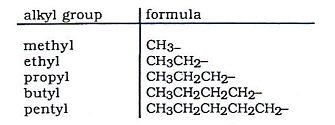• As well open chained alkanes, it is also possible for alkane molecules to form rings.
• These rings are called cyclo-alkanes, and have the same general formula as an alkene CnH2n. They have two less hydrogens, because there are no CH3 groups at the end of the chains.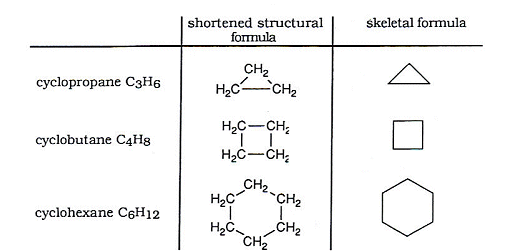• Every corner on the skeletal formula represents a CH2 group. Skeletal diagrams are very useful when drawing cyclic hydrocarbons.
• Skeletal diagrams can also be used to draw chained and branched isomers.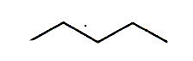• The above represents pentane, every kink in the line represents a CH2 group, and the ends represent a CH3 group.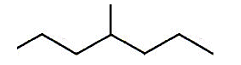• The above represents 4 methyl heptane.

## Shapes of Alkanes

• Representing three dimensional shapes in 2D on paper can give a misleading image of what the molecule looks like.
• The pairs of electrons in covalent bonds repeal each other and so arrange themselves around the carbon atom as far apart as possible.
• The C-H bonds in methane are directed so that they form a tetrahedron shape, with bond angles of 109o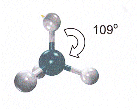• The structure of ethane: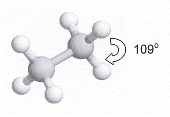• The structure of butane: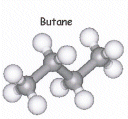• Hydrocarbon chains are not really straight, but are "Zig-Zaggged". All the bond angles are 109o

# Alkane characteristics

• What an alkane looks like at room temperature depends upon its size.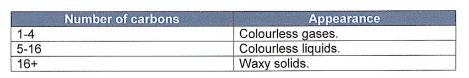• Alkanes contain non-polar molecules and mix well with each other; however they do not mix well with non-polar substances such as water.
• Alkanes are unreactive towards many laboratory reagents; they are unaffected by acids, alkalis, metals and oxidising agents.
• When they do react, it is usually in the gas phase, and energy is needed to get the reaction started.

## Melting and Boiling points of Alkanes

• As the molecular number increases, the boiling and melting point of the alkane increases.• C1 to C4 are gases, C5 to C17 are liquids, C18 and higher are solids.
• The increase in the boiling point is due to the stronger intermolecular forces.
• In hydrocarbons the only intermolecular forces are instantaneous dipole, induced dipole interactions.
• In a molecule, electrons are mobile, and at one point they may all find themselves at one end of the molecule. This will form a temporary dipole, affecting neighbouring molecules, pulling the molecules together.
• At the next instant the electrons could end up at the other side reversing the polarity of the molecule.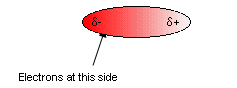• This permanent moving around of the electrons causes rapidly fluctuating dipoles which hold together the hydrocarbons.
• The higher the molecular weight of the compound, the more electrons there are in the molecules, meaning the stronger these Van der Waahl forces. This is why the melting points and boiling points of the larger molecules are higher.
• Branched alkanes have a lower Van der Waahl force than chains, due to their shape, which doesn’t allow the molecules get close enough to each other.

## Oxidation of Alkanes

• Alkanes do not react readily with air, but if they are heated they can combust to form carbon dioxide and water.
• The reaction has a high activation energy; the energy must be supplied in order for the reaction to begin.
• Alkanes must be vaporised before they can combust, thus more volatile hydrocarbons ignite easier.
• Once ignited, the reaction is very exothermic, which is why alkanes are often used for fuels.
• If the air supply is limited, the combustion may be incomplete, producing carbon dioxide and soot, along with partially combusted hydrocarbons.

# Molecular and Empirical Formula of Alkanes

• A molecular formula shows how many atoms of each type are present in a given compound.
• The empirical formula shows the smallest ratio of the different types of atom present.
• The Molecular Formula = M x Empirical Formula (where M= a positive integer).
• The empirical formula of a compound can be worked out from its percentage composition. For a hydrocarbon this can be found out by burning a known mass in oxygen and working out the amounts of carbon dioxide and water formed. This is called combustion analysis.
Example:

0.1g of a hydrocarbon x gave 0.309g CO2 and 0.142g H2O on combustion. What is the empirical formula of x?

Calculate the mass of C and H in 0.1g of the compound (all of the compound goes to H2O and CO2, so the mass of carbon and hydrogen in the compound can be worked out from the mass's of carbon dioxide and water produced).

44g CO2 contains 12g of C (Mr of CO2 is 44, and Ar of C is 12).
so 0.309g contains 0.309 x 12/44 = 0.0843g

18g H2O contains 2g of H
so 0.142g contains 0.142 x 2/18 = 0.0158g

So the compound contains 0.0843g of Carbon and 0.0158g of Hydrogen.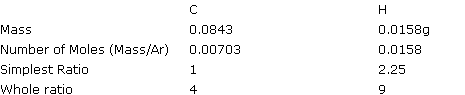So the empirical formula of x is C4H9.

## Useful books for revision:

Revise AS Chemistry for Salters (Written by experienced examiners and teachers of Salter's chemistry)Revise AS Chemistry for Salters (OCR) (Salters Advanced Chemistry)Home